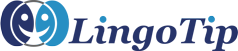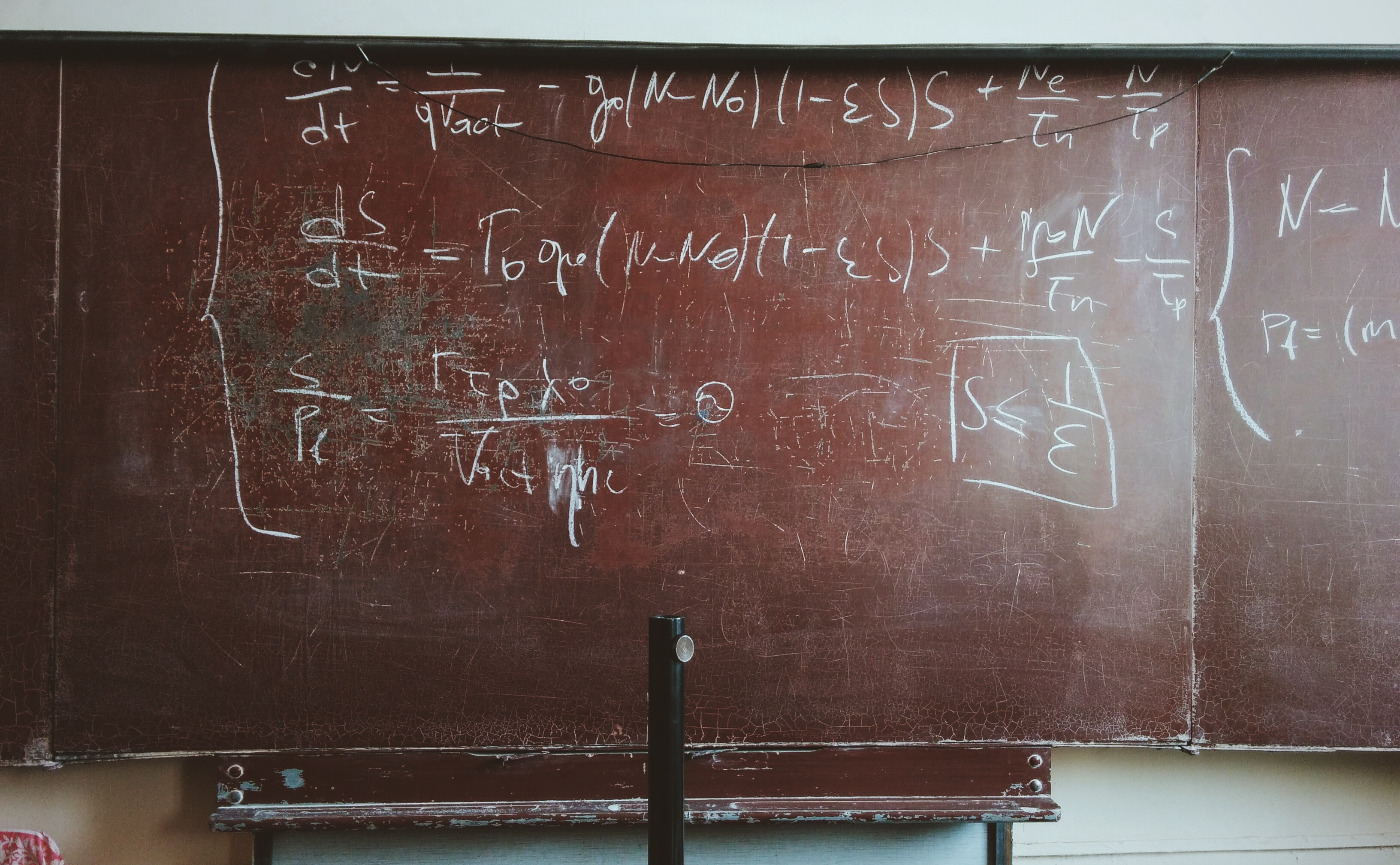# The Language of Mathematics

### Recent Articles

#### The Art of Rhetoric

A language is a set of symbols through which meaningful messages can be conveyed. A language can be defined as having unique symbols, with unique syntax rules for those symbols, which can be documented through writing and can be used to represent meaning in a specific context.

If this is the definition of language, then mathematics could also be defined as a language. Although mathematics is not a language like the English language, it certainly helps to organize ideas in people’s minds. It includes at least as many symbols as any other spoken language, with its own syntax rules and unique vocabulary.

Mathematics also borrows and lends words from and to other languages, such as the word radius from Latin and the words ellipse and parabola from Greek. The word triangle that defines a mathematical entity, has been borrowed by spoken languages to describe human relationships or a turning of 180-degree to describe the reversal of opinions.

Even the difficulty of acquiring the language of mathematics is similar to the difficulty of acquiring a foreign language. Indeed, there are parallels between the language of mathematics and the spoken language. But math is not a language in the usual sense of the word. One cannot say in “mathematics” sentences, such as “the sun is shining today” or “I love you” as can be said in any existing natural language. It might be more correct to define mathematics as a jargon similar to the jargon physicians use in their borrowed Latin or Greek jargon for medical terms. In this same way the mathematics jargon is used to describe many important aspects of our physical and theoretical world as a toolset of thought, but not beyond that.

The common perception is that mathematics is an accurate and clear language. However, mathematics has imaginative and obscure aspects as well. For example, have a look at the following problem:

The sum of an even combinations of the arithmetic series below is “0” and the sum of an odd combinations is “1”.

1-1+1-1+1-1+1-1=0
1-1+1-1+1-1+1-1+1=1

The question that mathematicians ask is what happens in the infinity?

a.  [1-1]+[1-1]+[1-1]+[1-1]+… => 0
b.  1+[-1+1]+[-1+1]+[-1+1]+[-1+1]+… => 1

Simplifying the problem by placing parentheses [1-1] as shown in the example (a) above, the sum appears to be “0”. And when placing the parentheses in this form [-1 + 1], as appears in example (b) above, the sum seems to lead to “1”.

In the early 18th century, this simple problem confused mathematicians and triggered a heated exchange of letters among them. Leibniz suggested that since the length of the infinite series is not even nor odd, the solution cannot be 0 or 1, but maybe their average, maybe ½. And so it was also accepted by other mathematicians that the amount of this infinite series is ½ even though the result, ½, looks impossible because it must either be 1 or 0. This demonstrates areas in mathematics where deduction is clear and precise compared to areas where mathematicians start speculating. And what Leibniz actually did is project the concept of mathematical addition from the finite to the infinite, theorizing the sum of infinite addition of that series to be equal to ½ when practical familiar tools are absent. Mathematicians, here, reject a formal result while forming a theoretic hypothesis.

There is a common joke about mathematicians that comes in a range of versions. Here it is told in the hot air balloon version. Two people flew in a hot air balloon and were lost. They spotted a man walking below them and shouted to him, “Where are we?” The man answered, “In a hot air balloon”. Following his reply, one of the people in the hot air balloon told his friend: “The man below is probably a true mathematician”. And when asked how he knew it, he explained “For two reasons: First because his answer is completely accurate, and second, because it doesn’t help us at all.”

This joke at the expense of mathematicians has some truth to it. When theoretical mathematics is a pure analytical inference it does not really bring new information about the world, and theoretically any person with mathematical knowledge may be able deduce these analytical inferences. However, as factual information is added, new information about the world is introduced.

To illustrate this, let’s take a look at the famous deductive argument:

Assumption A: All humans are mortal.
Assumption B: Socrates is human.
Conclusion: Socrates is mortal.

The conclusion, here, stems from the assumptions without a need for additional knowledge. The conclusion is true but does not add information that is not already included in the assumptions. Einstein said about geometry that the more it is certain, the less it is factual and the more it is factual, the more uncertain it becomes.

Analytical (deduction) does not provide new information. One must imagine, speculate and make assumptions making use of knowledge that comes from experience, to reach new information. This kind of thought is not analytical, it is not necessarily true, but it adds knowledge and development.

Here is an example of a non-analytical deduction:

Assumption A: Socrates is human.
Assumption B: Socrates is mortal.
Assumption C: All humans we have met so far are mortal.
Conclusion: All humans are mortal.

This argument is not necessarily true even though we all use it, generalizing, in the languages we speak and use such arguments all the time. And this is exactly the difference between the language of mathematics, which is purely analytical and the human language that incorporates generalizations and hypotheses that may not be true but potentially add to knowledge about the world.

In the city of Koenigsberg, in today’s Russia, there were seven bridges connecting the various parts of the city. Among the city’s residents the following conundrum has emerged: Would it be possible to cross all the bridges without crossing a bridge more than once? For a long time, the city’s residents tried to solve the problem, until in 1735 when the mathematician Leonard Euler proved that such a path is not possible. Euler’s solution to the Koenigsberg’s bridge problem was based on the mathematical Graph Theory and is considered the first practical solution using the mathematical Graph Theory. In this case, too, even though mathematics has a practical application, mathematics does not really add to knowledge about the world.

Mathematics has a great influence on the natural sciences, such as physics, biology, chemistry and even on economics, but very little influence on humanities – assuming, perhaps, that the humanities cannot be quantified by mathematics.

What is interesting, however, is that there are connections between mathematics and the evolution or change of natural languages ​​over time. In English, for example, the past verb is usually formed by adding “ed” to its end. For example, the verb walk in the present as opposed to walked in the past. However, there are other, irregular verbs, such as think versus thought. It turns out that irregular verbs change to regular verbs throughout history, and a study of more than one hundred irregular verbs and their use since the 12th-century revealed a mathematical trend in the historical change of the language. The study (by Jean-Baptiste Michel) found that the more that the irregular verb is used (e.g., 100 times more common), the slower it changes to become a regular verb at a scale of 10 times slower. This is, in fact, a mathematical analysis of historical change of language.

It is estimated that since ancient times, about 130 million books have been written, of which 20 million books have already been digitized by Google. The digitization trend of literature in general enables mathematical research to be carried out on that historical content. It is likely that in the future additional mathematical phenomena will be discovered related to the field of humanities in general and in languages ​​in particular.

* The joke and the sample arguments are brought from the book “Two Carriages and a Hot Air Balloon” by Michael Avraham.Photo by Roman Mager